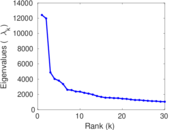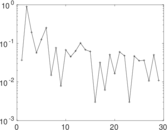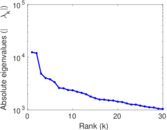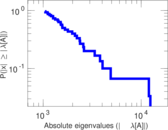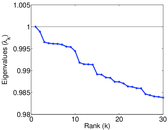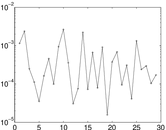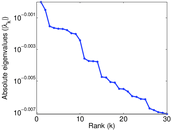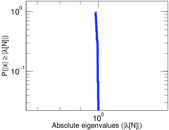# CiteULike user–tag

This is the bipartite network of users and tags in CiteULike. Each edge represents a tag assignment that connects a user and a tag. Since a user can assign a tag to multiple publications, the network contains multiple edges. The edges are annotated with the creation time of the tag assignment.

 Code `Cut` Internal name `citeulike-ut` Name CiteULike user–tag Data source http://www.citeulike.org/faq/data.adp AvailabilityDataset is available for download Consistency checkDataset passed all tests Category Interaction network Dataset timestamp 2007 Node meaning User, tag Edge meaning Assignment Network formatBipartite, undirected Edge typeUnweighted, multiple edges Temporal dataEdges are annotated with timestamps

## Statistics

 Size n = 175,992 Left size n1 = 22,715 Right size n2 = 153,277 Volume m = 2,411,819 Unique edge count m̿ = 538,761 Wedge count s = 192,371,639 Claw count z = 194,428,758,411 Cross count x = 302,871,675,261,597 Square count q = 146,940,165 4-Tour count T4 = 1,946,141,506 Maximum degree dmax = 189,295 Maximum left degree d1max = 57,706 Maximum right degree d2max = 189,295 Average degree d = 27.408 3 Average left degree d1 = 106.177 Average right degree d2 = 15.735 0 Fill p = 0.000 154 741 Average edge multiplicity m̃ = 4.476 60 Size of LCC N = 174,060 Diameter δ = 12 50-Percentile effective diameter δ0.5 = 3.609 05 90-Percentile effective diameter δ0.9 = 5.233 56 Median distance δM = 4 Mean distance δm = 4.262 34 Gini coefficient G = 0.912 555 Balanced inequality ratio P = 0.099 462 3 Left balanced inequality ratio P1 = 0.128 030 Right balanced inequality ratio P2 = 0.126 018 Relative edge distribution entropy Her = 0.827 610 Power law exponent γ = 2.821 69 Tail power law exponent γt = 1.791 00 Degree assortativity ρ = −0.097 101 6 Degree assortativity p-value pρ = 0.000 00 Spectral norm α = 12,418.1 Algebraic connectivity a = 0.031 527 5 Spectral separation |λ1[A] / λ2[A]| = 1.037 44 Controllability C = 142,640 Relative controllability Cr = 0.810 491

## Plots

### Fruchterman–Reingold graph drawing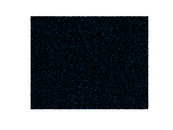### Degree distribution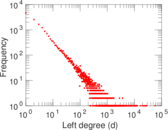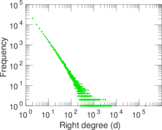### Cumulative degree distribution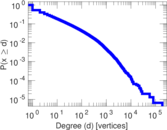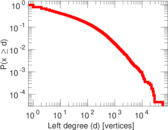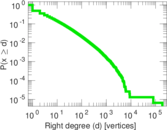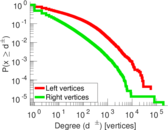### Lorenz curve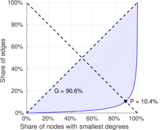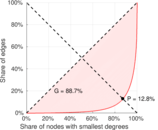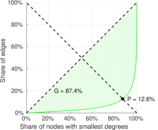### Spectral distribution of the adjacency matrix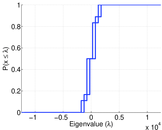### Spectral distribution of the normalized adjacency matrix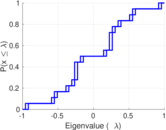### Spectral distribution of the Laplacian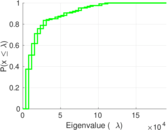### Spectral graph drawing based on the adjacency matrix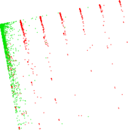### Spectral graph drawing based on the Laplacian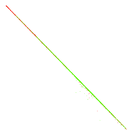### Spectral graph drawing based on the normalized adjacency matrix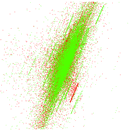### Degree assortativity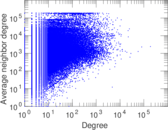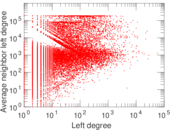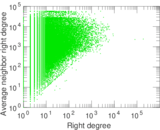### Zipf plot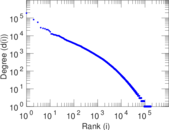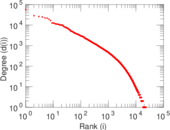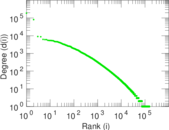### Hop distribution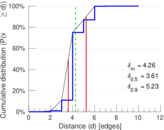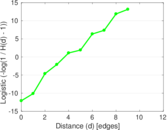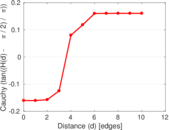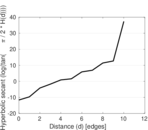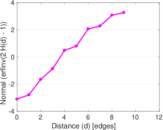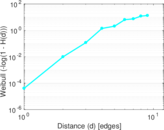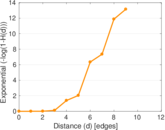### Delaunay graph drawing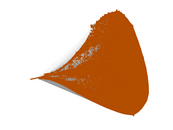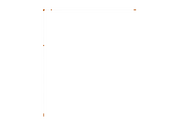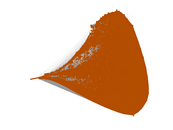### Edge weight/multiplicity distribution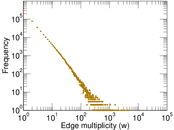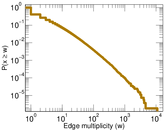### Temporal distribution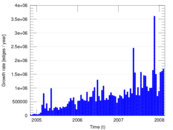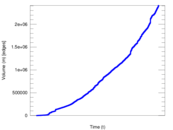### Diameter/density evolution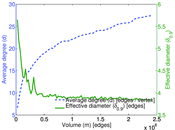### Inter-event distribution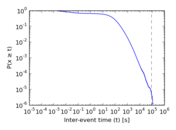### Node-level inter-event distribution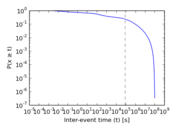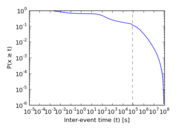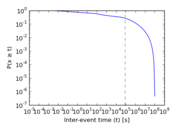### Matrix decompositions plots